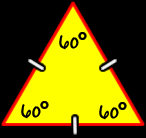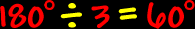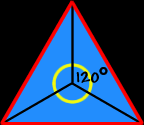# Polygons - Triangles

Properties of triangles, interior angles of triangles

 Polygons:  Properties of TrianglesSum of the Angles of a Triangle: The sum of the angles of a triangle is 180 degrees.ALWAYS!Regular Triangles - Equilateral Triangles: The properties of equilateral triangles:All sides are the same length (congruent) and all interior angles are the same size (congruent).To find the measure of the interior angles, we know that the sum of all the angles is 180 degrees (from above)...  And there are three angles...So, the measure of the interior angle of an equilateral triangle is 60 degrees.The measure of the central angles of an equilateral triangle:To find the measure of the central angle of an equilateral triangle, make a circle in the middle...  A circle is 360 degrees around...  Divide that by three angles...So, the measure of the central angle of an equilateral triangle is 120 degrees.